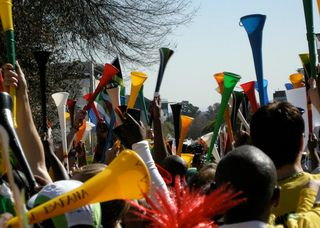Home Practice
For learners and parents For teachers and schools
Textbooks
Full catalogue
Pricing SupportLog in

We think you are located in United States. Is this correct?

# 10.4 Intensity of sound [NOT IN CAPS]

## 10.4 Intensity of sound [NOT IN CAPS] (ESADE)

Intensity is one indicator of amplitude. Intensity is the energy transmitted over a unit of area each second.

The unit of intensity is the decibel (symbol: dB).

 Source Intensity (dB) Times greater than hearing threshold Rocket Launch 180 $$\text{10}^{\text{18}}$$ Jet Plane 140 $$\text{10}^{\text{14}}$$ Threshold of Pain 120 $$\text{10}^{\text{12}}$$ Rock Band 110 $$\text{10}^{\text{11}}$$ Factory 80 $$\text{10}^{\text{8}}$$ City Traffic 70 $$\text{10}^{\text{7}}$$ Normal Conversation 60 $$\text{10}^{\text{6}}$$ Library 40 $$\text{10}^{\text{4}}$$ Whisper 20 $$\text{10}^{\text{2}}$$ Threshold of hearing 0 0

Table 10.3: Examples of sound intensities.

Vuvuzelas feature prominently at soccer events in South Africa. The intensity of the sound from a vuvuzela depends on how close you are. In Table 10.4 you can see how the intensity differs.

 Frequency (Hz) At ear Bell opening $$\text{1}$$ $$\text{m}$$ $$\text{2}$$ $$\text{m}$$ 125 36 62 38 35 250 92 106 82 85 500 103 121 102 101 1 000 106 122 108 100 2 000 101 122 110 101 4 000 97 109 110 102 5 000 93 111 109 100 8 000 87 110 107 98

Table 10.4: Average vuvuzela intensity measurements across frequencies at 4 distinct distances from the bell end of the vuvuzela (dBA) taken from South African Medical Journal (Cape Town, South Africa) 100 (4): 192

Vuvuzelas in actiontemp text